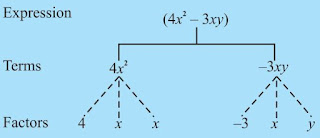# Chapter 12 Algebraic Expressions Class 7 Notes Maths

Chapter 12 Algebraic Expressions Class 7 Notes Maths is given on this page which are very helpful in understanding the various factors through which one can improve their efficiency and eventually scores higher in the exam. Through the help of Revision Notes for Class 7, student can arrange information in well manner that will make learning easier and more understandable. It will make you aware of the all the topics and ensure consistency in the preparation level. Also, you can find NCERT Solutions for Class 7 Chapter 12 Maths that will set a good foundation for your future goals.• Algebraic expression is formed from variables and constants using different operations.

• Symbols which are used to represent or replace numbers. They are denoted as x,y,z,a,b,c.... and can take different numerical values. We generally use small letters to represent variables.

• The parts of an expression which are formed separately first and then added are known as terms. Terms are added to form expressions.• Coefficient is the numerical factor in a term. Sometimes, any factor in a term is called the coefficient of the remaining part of the term.

• The terms having the same algebraic factors are called like terms.

• The terms having different algebraic factors are called unlike terms

Types of Algebraic Expressions

• Expression with one term is called a Monomial.

• Expression with two unlike terms is called a Binomial.

• Expression with three unlike terms is called a Trinomial.

• In general, an expression with one or more than one term is called a ‘Polynomial’. Thus a monomial, a binomial and a trinomial are all polynomials.

Addition and Subtraction of Algebraic Expressions

• The sum of two like terms is a like term with coefficient equal to the sum of coefficients of the two like terms.

• When we add two algebraic expressions, the like terms are added and the unlike terms are written as they are.

• The difference of two like terms is a like term with coefficient equal to the difference of coefficients of the two like terms.

• When we subtract two algebraic expressions, the like terms are subtracted and the unlike terms are written as they are.